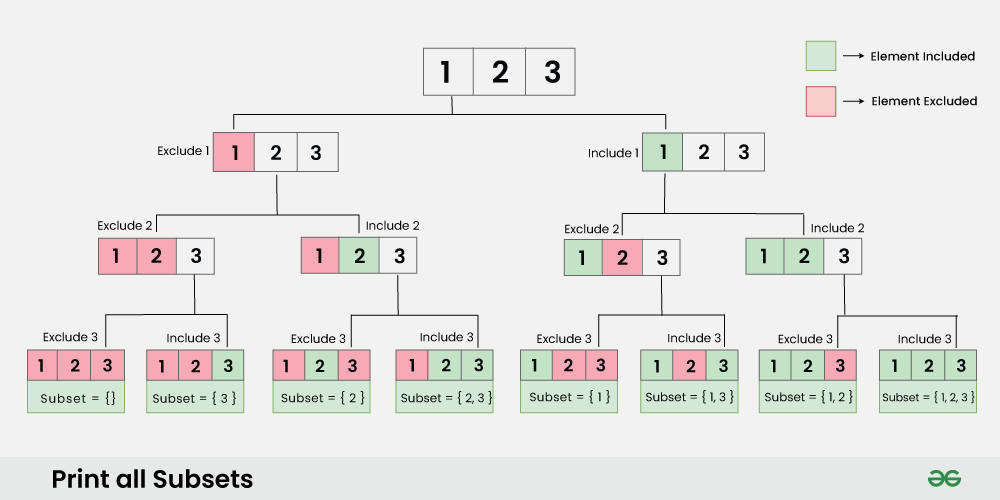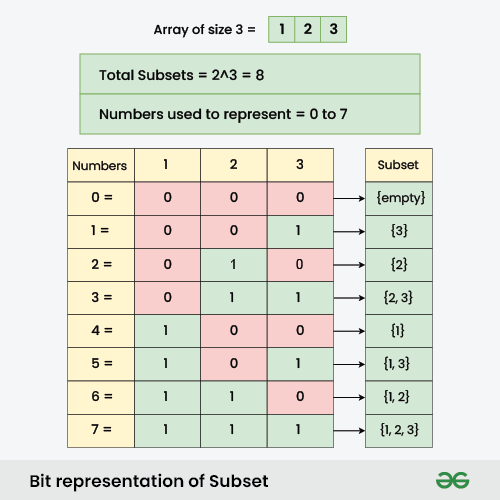Open In App
Related Articles

# Print all subsets of a given Set or Array

Given an array Arr[] of size N, print all the subsets of the array.

Subset: A subset of an array is a tuple that can be obtained from the array by removing some (possibly all) elements of it

Example:

Input: N = 3, Arr = [1, 2, 3]
Output: {}
{1}
{1, 2}
{1, 2, 3}
{1, 3}
{2}
{2, 3}
{3}
Explanation: These are all the subsets that can be formed from the given array, it can be proven that no other subset exists other than the given output.

Input: N = 2, Arr = [2, 4]
Output: {}
{2}
{2, 4}
{4}
Explanation: These are all the subsets that can be formed from the given array, it can be proven that no other subset exists other than the given output.

## How many Subsets are possible for an Array of size ‘N’ ?

Before jumping into the solution, can we observe some kind of relation between the size of array N and the number of subsets formed by that array? The answer is YES, there exists a relation that is given by the following formula:

Number of Subsets of an array of size N =Proof: For each element of the array we have 2 choices:

• Choice 1: Include it into the subset.
• Choice 2: Exclude it from the subset.

Since, each element has 2 choice to contribute into the subset and we have total N elements, therefore total subsets =Let’s see how can we construct our solution from this observation.

## Printing all subsets using Backtracking:

As mentioned above, for each element there two options, either include it into subset or exclude it. Backtracking algorithm can allow us to explore all possible choices one by one recursively.

### State Space Tree for printing all subsets using Backtracking:

Suppose an array of size 3 having elements {1, 2, 3}, the state space tree can be constructed as below:Follow the the steps below to implement the above idea:

• It starts with an empty subset and adds it to the result list.
• It iterates through the elements of the input vector:
• Includes the current element in the subset.
• Recursively calls itself with the updated subset and the next index.
• Excludes the current element from the subset (backtracks).

Below are the implementation of the above approach:

## C++

 `#include ``#include ``using` `namespace` `std;` `void` `calcSubset(vector<``int``>& A, vector >& res,``                ``vector<``int``>& subset, ``int` `index)``{``    ``// Add the current subset to the result list``    ``res.push_back(subset);` `    ``// Generate subsets by recursively including and``    ``// excluding elements``    ``for` `(``int` `i = index; i < A.size(); i++) {``        ``// Include the current element in the subset``        ``subset.push_back(A[i]);` `        ``// Recursively generate subsets with the current``        ``// element included``        ``calcSubset(A, res, subset, i + 1);` `        ``// Exclude the current element from the subset``        ``// (backtracking)``        ``subset.pop_back();``    ``}``}` `vector > subsets(vector<``int``>& A)``{``    ``vector<``int``> subset;``    ``vector > res;``    ``int` `index = 0;``    ``calcSubset(A, res, subset, index);``    ``return` `res;``}` `// Driver code``int` `main()``{``    ``vector<``int``> array = { 1, 2, 3 };``    ``vector > res = subsets(array);` `    ``// Print the generated subsets``    ``for` `(``int` `i = 0; i < res.size(); i++) {``        ``for` `(``int` `j = 0; j < res[i].size(); j++)``            ``cout << res[i][j] << ``" "``;``        ``cout << endl;``    ``}` `    ``return` `0;``}`

## Java

 `import` `java.util.ArrayList;``import` `java.util.List;` `public` `class` `Subsets {``    ``public` `static` `void` `calcSubset(List A,``                                  ``List > res,``                                  ``List subset,``                                  ``int` `index)``    ``{``        ``// Add the current subset to the result list``        ``res.add(``new` `ArrayList<>(subset));` `        ``// Generate subsets by recursively including and``        ``// excluding elements``        ``for` `(``int` `i = index; i < A.size(); i++) {``            ``// Include the current element in the subset``            ``subset.add(A.get(i));` `            ``// Recursively generate subsets with the current``            ``// element included``            ``calcSubset(A, res, subset, i + ``1``);` `            ``// Exclude the current element from the subset``            ``// (backtracking)``            ``subset.remove(subset.size() - ``1``);``        ``}``    ``}` `    ``public` `static` `List >``    ``subsets(List A)``    ``{``        ``List subset = ``new` `ArrayList<>();``        ``List > res = ``new` `ArrayList<>();` `        ``int` `index = ``0``;``        ``calcSubset(A, res, subset, index);` `        ``return` `res;``    ``}` `    ``// Driver code` `    ``public` `static` `void` `main(String[] args)``    ``{``        ``List array = List.of(``1``, ``2``, ``3``);``        ``List > res = subsets(array);` `        ``// Print the generated subsets``        ``for` `(List subset : res) {``            ``for` `(Integer num : subset) {``                ``System.out.print(num + ``" "``);``            ``}``            ``System.out.println();``        ``}``    ``}``}`

## Python

 `def` `calcSubset(A, res, subset, index):``    ``# Add the current subset to the result list``    ``res.append(subset[:])` `    ``# Generate subsets by recursively including and excluding elements``    ``for` `i ``in` `range``(index, ``len``(A)):``        ``# Include the current element in the subset``        ``subset.append(A[i])` `        ``# Recursively generate subsets with the current element included``        ``calcSubset(A, res, subset, i ``+` `1``)` `        ``# Exclude the current element from the subset (backtracking)``        ``subset.pop()`  `def` `subsets(A):``    ``subset ``=` `[]``    ``res ``=` `[]``    ``index ``=` `0``    ``calcSubset(A, res, subset, index)``    ``return` `res`  `# Driver code``if` `__name__ ``=``=` `"__main__"``:``    ``array ``=` `[``1``, ``2``, ``3``]``    ``res ``=` `subsets(array)` `    ``# Print the generated subsets``    ``for` `subset ``in` `res:``        ``print``(``*``subset)`

## C#

 `using` `System;``using` `System.Collections.Generic;` `class` `Program {``    ``static` `void` `CalcSubset(List<``int``> A,``                           ``List > res,``                           ``List<``int``> subset, ``int` `index)``    ``{``        ``// Add the current subset to the result list``        ``res.Add(``new` `List<``int``>(subset));` `        ``// Generate subsets by recursively including and``        ``// excluding elements``        ``for` `(``int` `i = index; i < A.Count; i++) {``            ``// Include the current element in the subset``            ``subset.Add(A[i]);` `            ``// Recursively generate subsets with the current``            ``// element included``            ``CalcSubset(A, res, subset, i + 1);` `            ``// Exclude the current element from the subset``            ``// (backtracking)``            ``subset.RemoveAt(subset.Count - 1);``        ``}``    ``}` `    ``static` `List > Subsets(List<``int``> A)``    ``{``        ``List<``int``> subset = ``new` `List<``int``>();``        ``List > res = ``new` `List >();``        ``int` `index = 0;``        ``CalcSubset(A, res, subset, index);``        ``return` `res;``    ``}` `    ``// Driver code``    ``static` `void` `Main(``string``[] args)``    ``{``        ``List<``int``> array = ``new` `List<``int``>{ 1, 2, 3 };``        ``List > res = Subsets(array);` `        ``// Print the generated subsets``        ``foreach``(List<``int``> subset ``in` `res)``        ``{``            ``Console.WriteLine(``string``.Join(``" "``, subset));``        ``}``    ``}``}`

## Javascript

 `function` `calcSubset(A, res, subset, index) {``    ``// Add the current subset to the result list``    ``res.push([...subset]);` `    ``// Generate subsets by recursively including and excluding elements``    ``for` `(let i = index; i < A.length; i++) {``        ``// Include the current element in the subset``        ``subset.push(A[i]);` `        ``// Recursively generate subsets with the current element included``        ``calcSubset(A, res, subset, i + 1);` `        ``// Exclude the current element from the subset (backtracking)``        ``subset.pop();``    ``}``}` `function` `subsets(A) {``    ``const subset = [];``    ``const res = [];``    ``let index = 0;``    ``calcSubset(A, res, subset, index);``    ``return` `res;``}` `// Driver code``function` `main() {``    ``const array = [1, 2, 3];``    ``const res = subsets(array);` `    ``// Print the generated subsets``    ``for` `(let i = 0; i < res.length; i++) {``        ``console.log(res[i].join(``' '``));``    ``}``}` `// Call the main function``main();`

Output

```1
1 2
1 2 3
1 3
2
2 3
3
```

### Complexity Analysis:

• Time Complexity: O(2^N), where n is the size of given array.
• Auxiliary Space:
• O(N) : if we only print our subsets, there will at max N recursion stack
• O(2^N) : if we would store all the subsets we will need 2^N memory blocks to store each subset

## Printing all Subsets Using Bit Manipulation

This approach is simpler compared to backtracking, as it just requires basic knowledge of bits.

Observation: A bit can be either 0 or 1. What can we deduce from this?

Since, each element has only two choices i.e. either get included or get excluded. Assign these choices to a bit representation such that:
0 means Excluded
1 means Included
i’th bit represents i’th element of the array

Now let’s say, there are N elements in the array. This array will have 2^N subsets. These subsets can be uniquely expressed in form of Bit representation of number from 0 to (2^N)-1.

Example: If elements in an array of size 2 = {A, B}
All the subsets of this array form the bit representation of number from 0 to (2^2)-1 i.e. 0 to 3

0 = 00 => A excluded, B excluded => { empty }
1 = 01 => A excluded, B included => { B }
2 = 10 => A included, B excluded => { A }
3 = 11 => A included, B included=> { A, B }

### Illustration:

Suppose given an array of size 3 = {1, 2, 3}. Generate all the subsets using bit manipulation as shown in the image below:Below are the step-by-step approach:

• Iterate numbers from 0 to (2^n)-1
• Generate binary representation of that number and include the elements of array as per below cases:
• If the i’th bit is 1, then include i’th element of the array into current subset
• If the i’th bit is 0, do nothing and continue.
• Each bit representation of the number will give a unique subset.

Below are the implementation of the above approach:

## C++

 `#include ``using` `namespace` `std;` `// Function to find the subsets of the given array``void` `findSubsets(``int` `nums[], ``int` `n)``{``    ``// Loop through all possible subsets using bit manipulation``    ``for` `(``int` `i = 0; i < (1 << n); i++) {` `        ``// Loop through all elements of the input array``        ``for` `(``int` `j = 0; j < n; j++) {` `            ``// Check if the jth bit is set in the current subset``            ``if` `((i & (1 << j)) != 0) {` `                ``// If the jth bit is set, add the jth element to the subset``                ``cout << nums[j] << ``" "``;``            ``}``        ``}` `        ``cout << endl;``    ``}``}` `// Driver Code``int` `main()``{``    ``int` `arr[] = { 1, 2, 3 };``    ``int` `n = ``sizeof``(arr)/``sizeof``(arr);``    ``findSubsets(arr, n);``}`

## Java

 `// Java program for the above approach` `import` `java.io.*;``import` `java.util.*;``class` `GFG {` `    ``// Function to find the subsets of``    ``// the given array``    ``public` `static` `void` `findSubsets(``int``[] nums)``    ``{``        ``// Get the length of the input array``        ``int` `n = nums.length;` `        ``// Loop through all possible subsets``        ``// using bit manipulation``        ``for` `(``int` `i = ``0``; i < (``1` `<< n); i++) {` `            ``// Loop through all elements``            ``// of the input array``            ``for` `(``int` `j = ``0``; j < n; j++) {` `                ``// Check if the jth bit is set``                ``// in the current subset``                ``if` `((i & (``1` `<< j)) != ``0``) {` `                    ``// If the jth bit is set,``                    ``// add the jth``                    ``// element to the subset``                    ``System.out.print(nums[j] + ``" "``);``                ``}``            ``}` `            ``System.out.println();``        ``}``    ``}` `    ``// Driver Code``    ``public` `static` `void` `main(String[] args)``    ``{``        ``int``[] arr = ``new` `int``[] { ``1``, ``2``, ``3` `};``        ``findSubsets(arr);``    ``}``}`

## Python3

 `#Function to find the subsets of the given array``def` `findSubsets(nums, n):``    ``# Loop through all possible subsets using bit manipulation``    ``for` `i ``in` `range``(``1` `<< n):``        ``# Loop through all elements of the input array``        ``for` `j ``in` `range``(n):``            ``#Check if the jth bit is set in the current subset``            ``if` `(i & (``1` `<< j)) !``=` `0``:``                ``#If the jth bit is set, add the jth element to the subset``                ``print``(nums[j], end``=``" "``)``        ``print``()``#Driver Code``arr ``=` `[``1``, ``2``, ``3``]``n ``=` `len``(arr)``findSubsets(arr, n)`

## C#

 `using` `System;` `public` `class` `GFG {``    ``// Function to find the subsets of the given array``    ``static` `void` `FindSubsets(``int``[] nums)``    ``{``        ``int` `n = nums.Length;` `        ``// Loop through all possible subsets using bit``        ``// manipulation``        ``for` `(``int` `i = 0; i < (1 << n); i++) {``            ``// Loop through all elements of the input array``            ``for` `(``int` `j = 0; j < n; j++) {``                ``// Check if the jth bit is set in the``                ``// current subset``                ``if` `((i & (1 << j)) != 0) {``                    ``// If the jth bit is set, add the jth``                    ``// element to the subset``                    ``Console.Write(nums[j] + ``" "``);``                ``}``            ``}` `            ``Console.WriteLine();``        ``}``    ``}` `    ``// Driver Code``    ``public` `static` `void` `Main(``string``[] args)``    ``{``        ``int``[] arr = { 1, 2, 3 };``        ``FindSubsets(arr);``    ``}``}` `// This code is contributed by shivamgupta0987654321`

## Javascript

 `// Function to find the subsets of the given array``function` `findSubsets(nums, n) {``    ``// Loop through all possible subsets using bit manipulation``    ``for` `(let i = 0; i < (1 << n); i++) {``        ``// Loop through all elements of the input array``        ``for` `(let j = 0; j < n; j++) {``            ``// Check if the jth bit is set in the current subset``            ``if` `((i & (1 << j)) !== 0) {``                ``// If the jth bit is set, add the jth element to the subset``                ``process.stdout.write(nums[j] + ``" "``);``            ``}``        ``}``        ``console.log();``    ``}``}` `// Driver Code``let arr = [1, 2, 3];``let n = arr.length;``findSubsets(arr, n);``// THIS CODE IS CONTRIBUTED BY YASH AGARWAL(YASHAGARWAL2852002)`

Output

```1
2
1 2
3
1 3
2 3
1 2 3
```

### Complexity Analysis:

• Time Complexity: O(2^N), where N is the size of given array.
• Auxiliary Space:
• O(1) : if we only print our subsets
• O(2^N) : if we would store all the subsets we will need 2^N memory blocks to store each subset